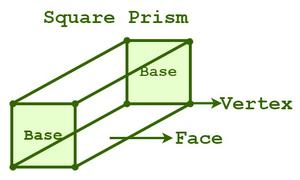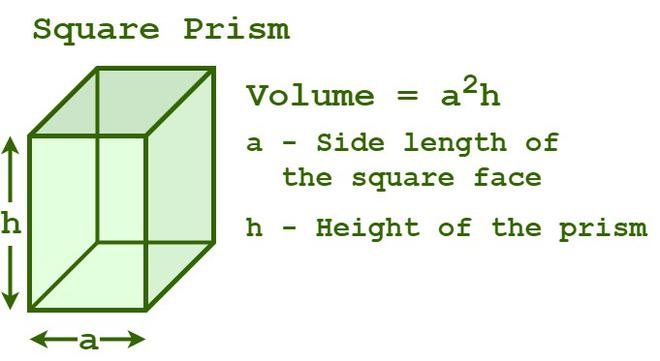Open in App
Not now

# Volume of a Square Prism

• Last Updated : 22 Aug, 2022

A square prism is a three-dimensional-shaped geometric structure that has two congruent square bases that are connected by four rectangular faces. A prism having a square base is defined as a square prism. All cubes fulfill all the properties of a square prism, so all cubes are square prisms, but not all square prisms are cubes. A square prism has a total of six faces (two square bases and four rectangular faces), twelve edges, and eight vertices. The lateral faces (or) sides faces are rectangles, and the two bases are squares, the area of the prism is the total area of all its faces. Depending upon the alignment of the square bases, a square prism is classified into two types, a right square prism, and an oblique square prism. Right square prisms have lateral faces that are perpendicular to their square bases, whereas oblique square prisms have lateral faces that aren’t perpendicular to the square bases.## Volume of Square Prism

The volume of a square prism is the total amount of space that is enclosed by the prism. The volume or capacity is in terms of cubic units and is equal to the product of the base area and the height.

Formula for calculating the volume of any prism is given as follows:

Volume of a prism (V) = Base area × Height of the prism

## Volume of Square Prism Formula

The formula for finding the volume of a square prism is base multiplied by area.

Now, the two congruent bases of the prism are squares.

Hence, the base area = (edge)2 = a2 square units

Now, substitute the values in the above formula, we get

Volume of a square prism = a2h cubic units

Where “a” is the side of the square base and, “h” is the height of the square prism.## How To Calculate the Volume of Square Prism?

Steps to Calculate the Volume of Square Prism are explained below:

Step 1:

Write the given dimensions of the square prism. Now s = side of square prism, s2 = area of the base, and h = height of square prism.
Make sure that the units of s and h should be the same.

Step 2:

Put these respective values in the volume of square prism formula s2h.

Step 3:

The value obtained is the volume of the square prism. Write the unit of the volume of the square prism is in terms of units3.

Consider the following example:

Example: Find the volume of a square prism if the edge of the base is 9 and its height is 11.

Solution:

Given data,

edge of base = 9

height of a square prism (h) = 11

We have,

The Volume of a square prism = Base area × height of the prism

By substituting the values in the formula, we get

= 92 × 11 = 891 cu. units

Therefore, the volume of a square prism is 891 cu. units.

## Solved Examples based on the Volume of a Square Prism

Example 1: Calculate the volume of a square prism whose side is 10 inches and whose height is 15 inches.

Solution:

Given data,

The length of the side of a square prism (a) = 10 inches

The height of a square prism (h) = 15 inches

We have,

The Volume of a square prism = a2h cubic units

= (10)2 × 15

= 100 × 15 = 1500 cu. in

Thus, the volume of the given prism is 1500 cu. in.

Example 2: Find the volume of a square prism if the length of the side of the square face of a square prism is 12 cm and its height is 17 cm.

Solution:

Given data,

The length of the side of a square prism (a) = 12 cm

The height of a square prism (h) = 17 cm

We have,

The Volume of a square prism = a2h cubic units

= (12)2 × 17

= 144 × 17 = 2448 cu. cm

Therefore, the volume of a square prism is 2448 cu. cm.

Example 3: Determine the volume of a square prism whose length of the side of the square face is 8 units and whose height is 10 units.

Solution:

Given data,

The length of the side of a square prism (a) = 8 units

The height of a square prism (h) = 10 units

We have,

The Volume of a square prism = a2h cubic units

Now, substitute the values of a and h in the formula.

= (8)2 × 10

= 64 × 10 = 640 cubic units

Thus, the volume of the given prism is 640 cubic units.

Example 4: Find the length of the edge of the square prism if the volume of the square prism is 1,694 cu. cm and the height is 14 cm.

Solution:

Given data,

The height of a square prism (h) = 14 cm

The volume of a square prism = 1694 cu. cm

We have,

The Volume of a square prism = a2h cubic units

By substituting the values in the formula, we get

⇒ 1694 = (a2) × (14)

⇒  a2 = 1694 ÷ 14 = 121

⇒ a = √121 = 11 cm

Thus, the length of the edge of the square prism is 11 cm.

## FAQs on Volume of a Square Prism

Question 1: What is the formula for finding the volume of a square prism?

Formula for calculating the volume of prism is given by V = (base area) × (height of the prism). A square prism is a prism whose base is in the shape of a square.

Volume of a square prism = a2h cubic units.

Here, “a” is the length of the side of the square face and “h” is the height of the square prism.

Question 2: What will happen to the volume of a square prism if the height of the square prism is doubled?

We know that the formula for calculating the volume of any prism is (base area) × (height of the prism). We can clearly say that the volume of the prism is directly proportional to its height. So, as the height of the square prism is doubled, its volume will also be doubled.

Question 3: What happens to the volume of a square prism if the length of the square face is reduced to half?

The formula for calculating the volume of a square prism.

V = a2h cubic units.

Here, “a” is the edge length of the square face, and “h” is the height of the square prism. Thus, the volume of the prism is directly proportional to the square of the length of edge of square face.

V’ = (a/2)2h = (a2h)/4 = V/4

So, the new volume of the square prism is one-fourth of its old volume.

Question 4: How can we find the height of a square prism if the volume of the square prism and the length of the edge of the base are given?